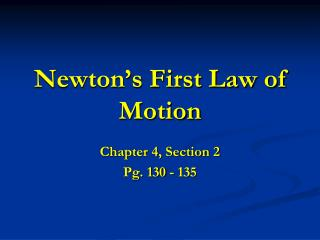DownloadDownload PresentationNewton’s First Law of Motion

# Newton’s First Law of Motion

Télécharger la présentation## Newton’s First Law of Motion

- - - - - - - - - - - - - - - - - - - - - - - - - - - E N D - - - - - - - - - - - - - - - - - - - - - - - - - - -
##### Presentation Transcript

1. Newton’s First Law of Motion Chapter 4, Section 2 Pg. 130 - 135

2. “An object at rest remains at rest, and an object in motion continues in motion with a constant velocity unless the object experiences a net external force.” Newton’s First Law of Motion (Inertia) Inertia is the tendency of an object to maintain its state of motion.

3. Fr Fx ΣF Forces Stationary Object An applied external force (Fx) greater than an object’s natural resisting force (Fr)will cause the object to move. The forces are added together to get the net force (ΣF).

4. Net external force is total force resulting from a combination of external forces on an object. When there is no change in motion, an object is said to be at equilibrium. Newton’s First Law of Motion (Cont.)

5. 5120 N 950 N 1520 N 4050 N F 1070 N θ 570 N Example Problem: Four forces act on a hot-air balloon (shown below). Find the magnitude and direction of the resultant force on the balloon. ∑ F in X direction 1520 N – 950 N = 570 N Left ∑ F in Y direction 5120 N – 4050 N = 1070 N Up F ² = (1070 N) ² + (570) ² F= 1212 N θ = Tan ־¹(1070 N / 570 N) θ = 62° up from the horizontal

6. ΣF ΣF Newton’ First Law of Motion (Cont.) - Mass is also a measurement of inertia. • The greater the mass of an object, the less it accelerates under an applied force. Therefore the greater mass has the greater inertia.